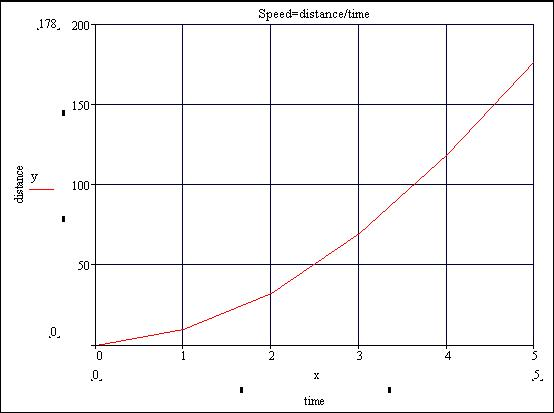# Velocity Problem

## Homework Statement

The position of a car is given by the values below.

Seconds | Feet
1 | 10
2 | 32
3 | 70
4 | 119
5 | 178

Find the average velocity for the time period beginning when t=2 and lasting
(a) 3 seconds
(b) 2 seconds
(c) 1 second

## Homework Equations

There were no equations provided

## The Attempt at a Solutioni usually have an equation to go by, but i do not in this case.
all attempts to make an equation out of the data have failed.
i think it is an exponential curve, but i can find the exact formula.
if anyone could stear me in the direction so i can figure this problem out.
i want to know what i'm doing, so do not give me the answer but please someone point me in some productive direction.

would i just input the numbers in the slope equation?
M=
y-y1
----
x-x1
i have the x and y already.
that seems too easy.

HallsofIvy
Homework Helper
xviddivxoggmp3, you are completely right- it is that easy. Shrodinger's dog is also correct but might be a bit confusing here. His point is that this problem is leading you to the idea of a "limit" and then the velocity at a single time.

xviddivxoggmp3, you are completely right- it is that easy. Shrodinger's dog is also correct but might be a bit confusing here. His point is that this problem is leading you to the idea of a "limit" and then the velocity at a single time.

Yeah I edited, I kind of misread it, but then I reread his question and saw how simple what he was asking was.Here's a graph I did,I had a bit of time and wanted a bit of practice.

Graph of:-

Seconds | Feet
1 | 10
2 | 32
3 | 70
4 | 119
5 | 178Essentially the question is asking you to find an exact point on the graph.

Last edited: